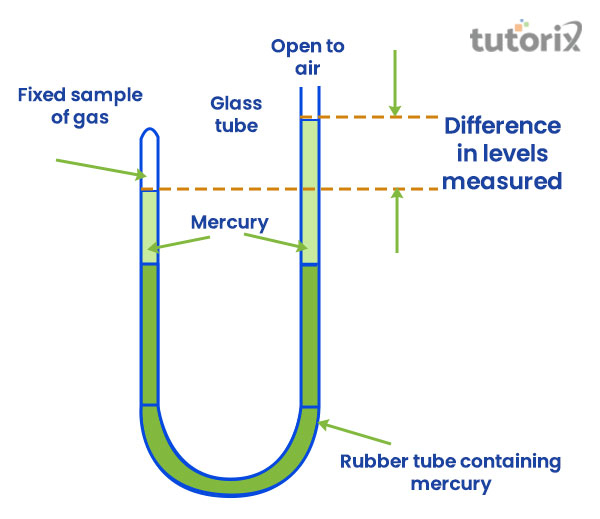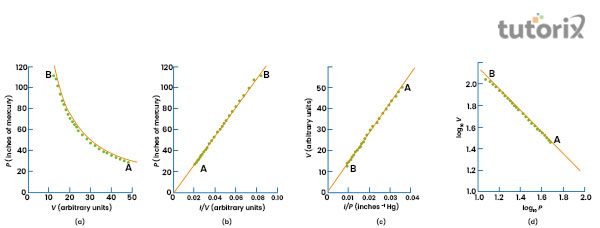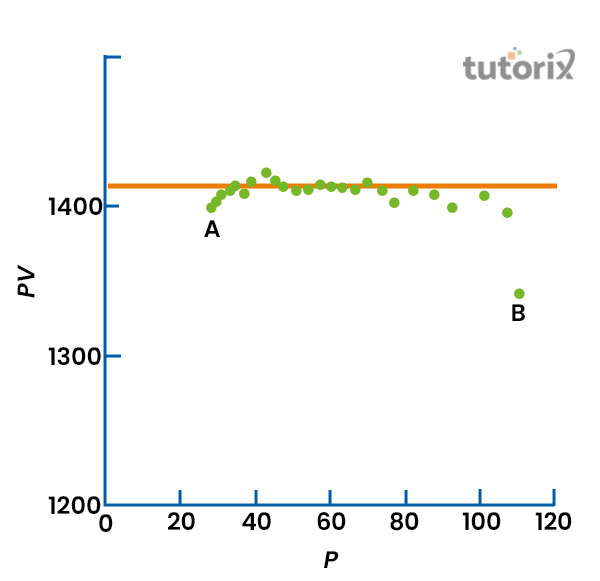# Boyles Law

## Introduction

Boyle's Law, which describes the effect of pressure on gas, is the most often utilised and has the most influence out of the three basic Gas Laws that exists. As a result, it will be the focus of this tutorial. The usage of scuba tanks by divers who need to operate and breathe underwater is a simple illustration of Boyle's Law in action in the real world.

How is it that a diver can stay submerged for so long inhaling from a scuba tank with such a little internal volume capacity which is usually less than percent cubic feet? The tank is inflated to between 4000 and 5000 psig, according on the answer. Because air is compressible, we can "try to squeeze" a lot of air into the tank by exerting a lot of pressure while the tank volume remains constant. Boyle's Law is a formula for calculating the volume change caused by a change in pressure.

## Statement for Boyle’s Law

Boyle's Law asserts that if we take a fixed volume of gas and increase the pressure placed on it, the molecules of gas will migrate closer together. Also, the gas would consume less space.

For example, the space required within the pipe systems in gas distribution and transmission systems is fixed and constant. As a result, raising system pressure would also increase the density of gas moving through the pipe network.

Hence, Boyle’s Law statement simplifies to

"The volume of an ideal gas decreases as increasing pressure is applied to it, at constant pressure."

## Derivation for Boyle’s Law

Boyle’s Law asserts that the volume, V, of a certain mass of gas varies inversely with its pressure, p, at constant temperature.

Hence, one could easily write, $\mathrm{V\:\varpropto\:\frac{1}{P}}$

or, $\mathrm{PV = k_1}$

From this equation, one could also write $\mathrm{P_1V_1 = P_2V_2}$

According to the above equation, the product of the initial volume and pressure is equal to the product of the volume and pressure following a change in one of them under constant temperature.

## Experimental Set Up for Boyle’s LawThe apparatus illustrated in the diagram is used to conduct an experiment to test Boyle's law. Raising or lowering the other end can change the volume and pressure of the gas (air) trapped in the closed end. The pressure of the gas in the closed end may be estimated by measuring the difference in mercury levels in the two tubes. Assuming the glass tube is a cylinder, the volume of gas in this end may be computed.

## Analysis of Data for Boyle’s Law

Consider the plots shown below corresponding to Boyle’s Law:Once the data from the above experiments have been obtained, one can attempt to decipher a mathematical relation using the mutually independent quantities that have been measured. One of methods could be to plot the various powers of different quantities measured against each other to get a straight line. The mathematical relation for straight line is

$$\mathrm{y\:=\:mx + c}$$

Wherein m and c are constants while x and y are variables. In the above figure one can observe plots of P versus V (Plot (a)) , P versus 1/V (Plot (b)) , V versus 1/P (Plot (c)) and log V versus log P (Plot (d)). The plots of P versus 1/V (Plot (b)) and V versus 1/P (Plot (c)) give straight line through the origin. The plot of log V vs log P also gives a straight line but it has a negative slope of -1. From these plots, one can deduce the following equations

$$\mathrm{P\:=\:\frac{m}{V}}$$

$$\mathrm{V\:=\:\frac{m}{P}}$$

$$\mathrm{and,\:log\:V\:=\:log\:m\:-\:log\:P}$$

If the mathematical relation between the two quantities is as simple as the ones measured in the experiment for establishing Boyle’s law, it is possible to derive them numerically as well. When the values of P are multiplied with corresponding values of V, the products would be approximately uniform for a particular gas at constant temperature. Thus, from the plots one could very well deduce:

$$\mathrm{PV\:=\:m}$$

The above equation represents hyperbola, which is obtained by plotting P and V as shown in plot (a).

## Conclusion

The gas laws discussed in this tutorial were established nearly four centuries ago, and they've helped scientists figure out how to calculate variables pressures, volumes, and temperatures of gases under different conditions. Under the assumption of constant temperature, Robert Boyle found the relationship between pressure and volume in 1662. If the temperature remains constant, the pressure exerted by the gas will decrease as the volume of the container expands.

## FAQs

Q1. State Boyle’s Law.

Ans: "The pressure of a gas is inversely proportional to the volume of the gas under constant temperature, for a given number of moles of a particular gas molecule."

Q2. hat would the PV versus P plot look like for a gas obeying Boyle’s Law?

Ans: From Boyle's Law, one could deduce the relation that the product of pressure and volume for a particular gas at constant temperature is a constant. Hence, the plot of PV versus P would give a horizontal straight line which does not pass through the origin.Q3. Mention some real life application of Boyle’s Law.

Ans: The real life application of Boyle’s Law are as follows:

(i) Exhale and Inhale are two separate processes in the mechanism of breathing or respiration, as we all know. As a result, when we inhale, the volume of our lungs expands, lowering the pressure inside the lungs in comparison to the outside atmosphere. Air rushes into the lungs as a result.

(ii) When we pump the piston of a syringe, the amount of liquid within the barrel decreases, resulting in an increase in liquid pressure. As a result, the fluid is administered into the body of the patient.

Q4. Write down the mathematical relation for Boyle’s Law.

Ans: The mathematical equation for Boyle’s Law can be represented as:

$$\mathrm{V\:\varpropto\:\frac{1}{P}}$$

$$\mathrm{or,\:PV\: =\: k_1}$$

where, P = Pressure of the gas, V = Volume of the gas,

$\mathrm{k_1}$ = Constant

Q5. Who stated Boyle’s Law?

Ans: Boyle’s Law was stated by English natural philosopher, Robert Boyle.

Updated on: 22-Aug-2023

349 Views